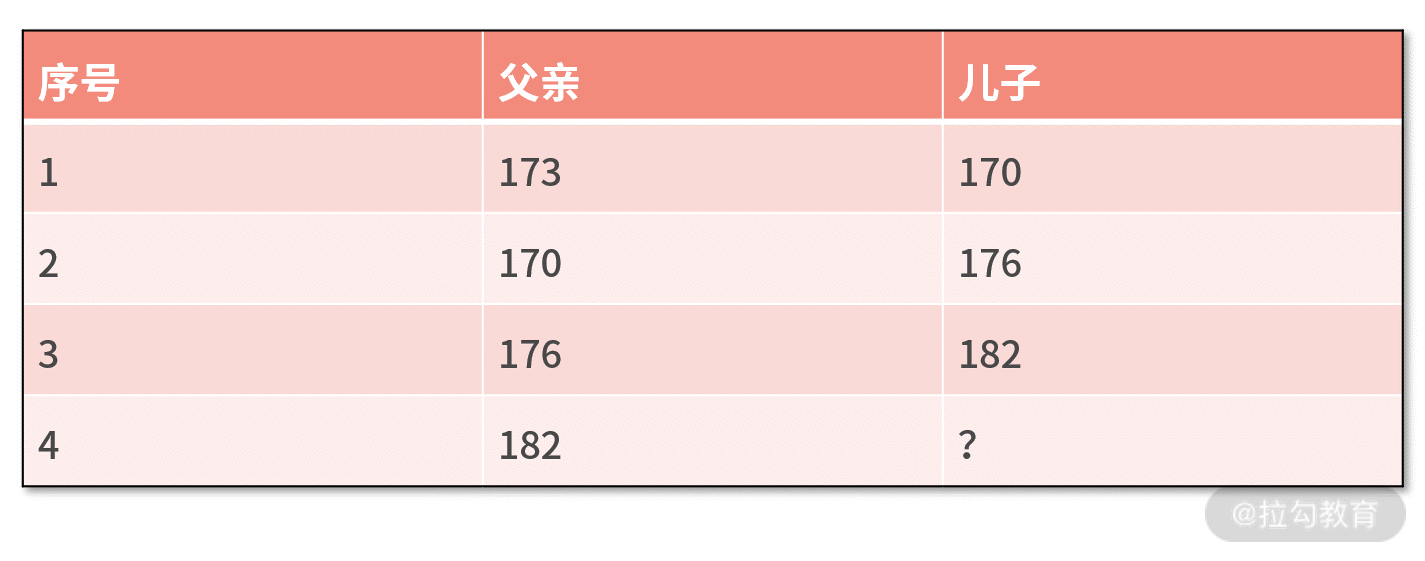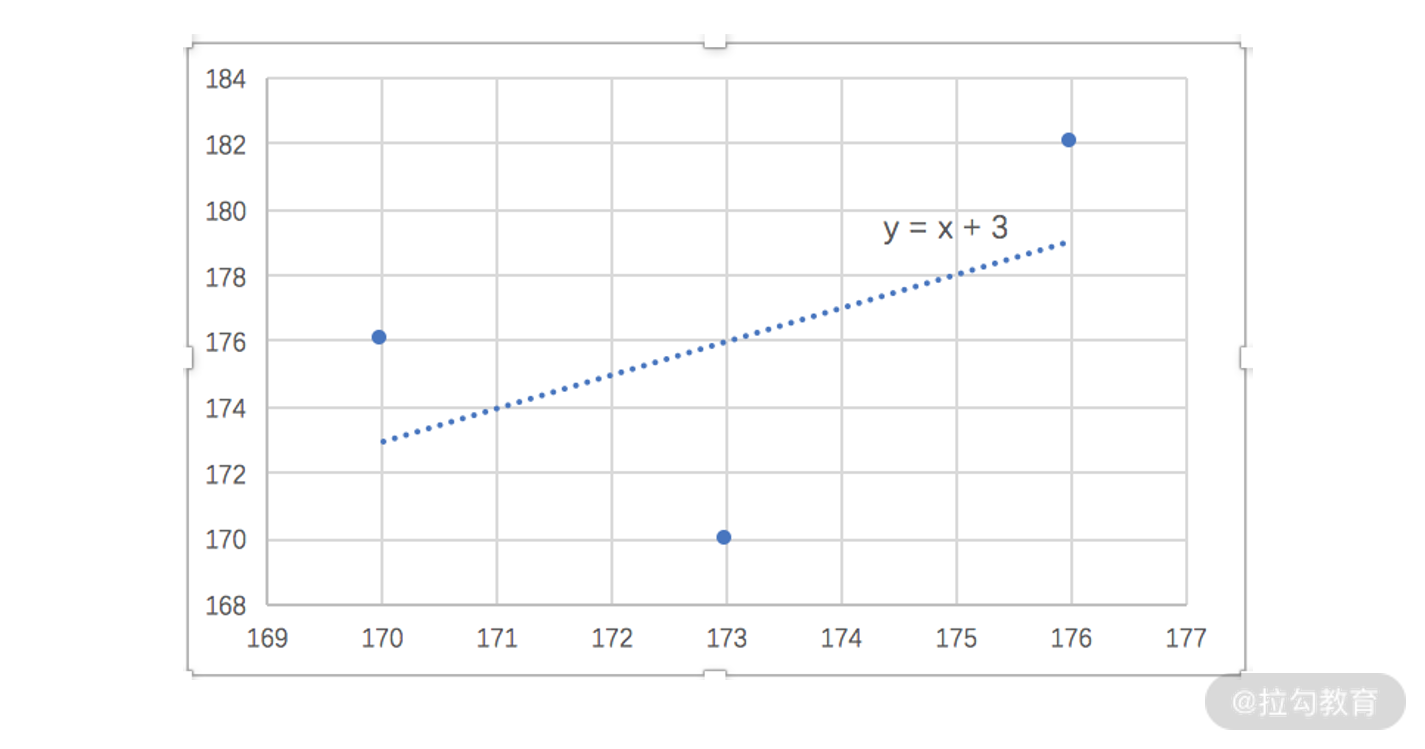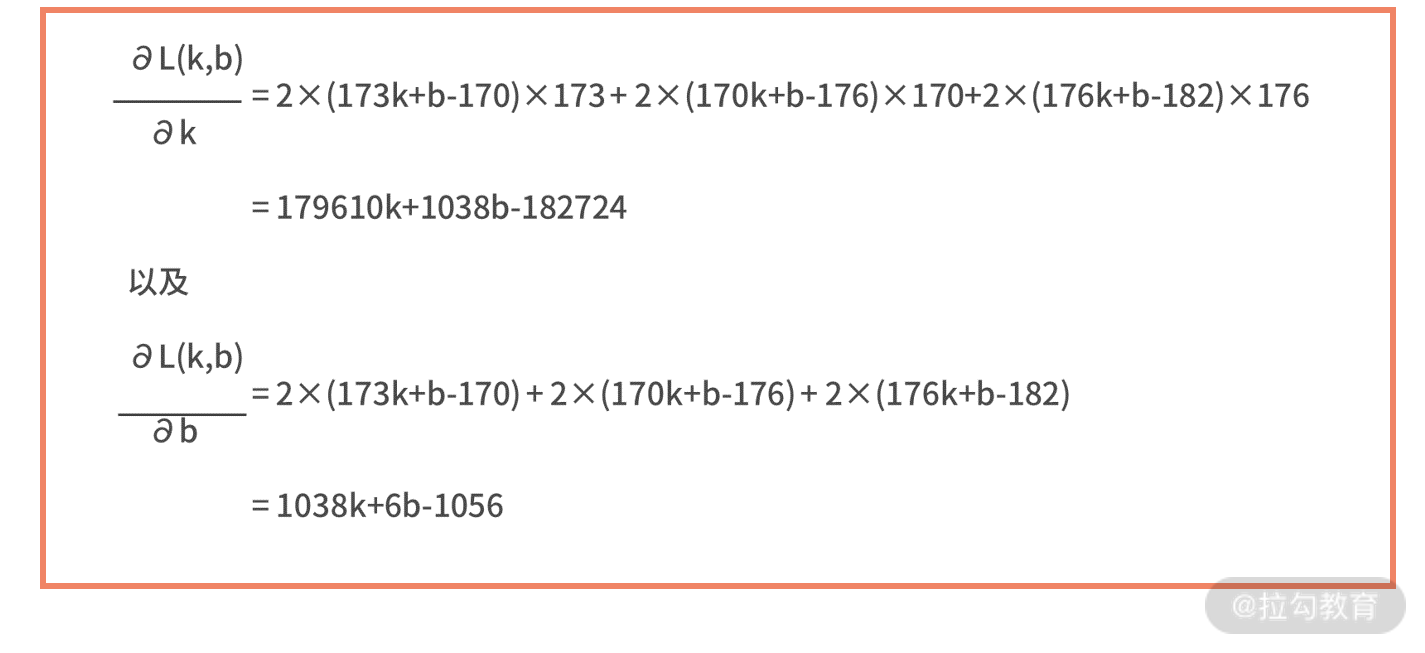18 AI 入门：利用 3 个公式搭建最简 AI 框架

从“身高预测”认识 AI 本质人工智能建模框架的三个公式

• 第一步，根据假设，写出模型的输入、输出关系 y = f(w; x)；
• 第二步，根据偏差的计算方法，写出描述偏差的损失函数 L(w)；
• 第三步，对于损失函数，求解最优的参数值，即w*= argmin L(w)。

• 第一步的假设，可以由线性模型调整为高阶多项式的假设 y=ax2+bx+c；
• 第二步的损失函数，可以由平方误差调整为绝对值求和的误差，即 L(w) = |y1 - ŷ1| + |y2 - ŷ2| + |y3 - ŷ3|；
• 第三步的求解最优，可以采用求导法，也可以调整为梯度下降法，甚至可以用一些启发式方法求解。

用 AI 基本框架重新看“线性回归”

• 第一步，根据假设，写出模型的输入、输出关系 y = f(w; x)。我们假设是线性模型，则有

y = kx + b。

• 第二步，根据偏差的计算方法，写出描述偏差的损失函数 L(w)。我们选择平方误差，则有

L(w) = (y1 - ŷ1)2 + (y2 - ŷ2)2 + (y3 - ŷ3)2。其中w= [k,b]，我们再把 y=kx+b 和三对父子的实际身高 xi、ŷi 代入上式，则有 L(k,b) = (173k+b-170)2 + (170k+b-176)2 + (176k+b-182)2。

• 第三步，对于损失函数，求解最优的参数值，即w*= argmin L(w)。为了求解函数的极小值，我们考虑计算损失函数关于 k 和 b 的导数，则有小结

• 第一步，根据假设，写出模型的输入输出关系 y = f(w; x)；
• 第二步，根据偏差的计算方法，写出描述偏差的损失函数 L(w)；
• 第三步，对于损失函数，求解最优的参数值，即w*= argmin L(w)。